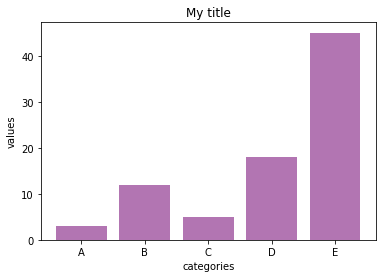In the following example, title, x label and y label are added to the barplot using the `title()`, `xlabel()`, and `ylabel()` functions of the matplotlib library.

Those functions are applied to a barplot in the example, but the same method would work for other chart types.

``````# libraries
import numpy as np
import matplotlib.pyplot as plt

# create dataset
height = [3, 12, 5, 18, 45]
bars = ('A', 'B', 'C', 'D', 'E')
x_pos = np.arange(len(bars))

# Create bars and choose color
plt.bar(x_pos, height, color = (0.5,0.1,0.5,0.6))

# Add title and axis names
plt.title('My title')
plt.xlabel('categories')
plt.ylabel('values')

# Create names on the x axis
plt.xticks(x_pos, bars)

# Show graph
plt.show()``````Note: the matplotlib section provides a lot of tips and tricks on how to customize a matplotlib chart!

## Contact & Edit

👋 This document is a work by Yan Holtz. You can contribute on github, send me a feedback on twitter or subscribe to the newsletter to know when new examples are published! 🔥